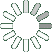Puzzle Command
Daily Puzzle
Number Logic #11
January 11, 2015Find a 6 digit number where:

The 1st digit is even.
The 2nd digit is even.
The 3rd digit is odd.
The 4th digit is odd.
The 5th digit is even.
The 6th digit is odd.

Every digit is larger than the previous digit.

Puzzle Notes
6 digit numbers range from 100,000 to 999,999.
Enter in your solution below and click submit.